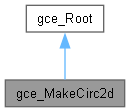# gce_MakeCirc2d Class Reference

This class implements the following algorithms used to create Circ2d from gp. More...

`#include <gce_MakeCirc2d.hxx>`

Inheritance diagram for gce_MakeCirc2d:[legend]

## Public Member Functions

gce_MakeCirc2d (const gp_Ax2d &XAxis, const Standard_Real Radius, const Standard_Boolean Sense=Standard_True)
The location point of XAxis is the center of the circle. Warnings : It is not forbidden to create a circle with Radius = 0.0 If Sense is true the local coordinate system of the solution is direct and non direct in the other case. The status is "NegativeRadius" if Radius < 0.0. More...

gce_MakeCirc2d (const gp_Ax22d &Axis, const Standard_Real Radius)
The location point of Axis is the center of the circle. Warnings : It is not forbidden to create a circle with Radius = 0.0. More...

gce_MakeCirc2d (const gp_Circ2d &Circ, const Standard_Real Dist)
Makes a Circ2d from gp <TheCirc> concentric with another circ2d <Circ> with a distance <Dist>. If Dist is greater than zero the result encloses the circle <Circ>, else the result is enclosed by the circle <Circ>. The local coordinate system of the solution is the same as Circ. More...

gce_MakeCirc2d (const gp_Circ2d &Circ, const gp_Pnt2d &Point)
Makes a Circ2d from gp <TheCirc> concentric with another circ2d <Circ> and passing through a Pnt2d <Point>. The local coordinate system of the solution is the same as Circ. More...

gce_MakeCirc2d (const gp_Pnt2d &P1, const gp_Pnt2d &P2, const gp_Pnt2d &P3)
Makes a Circ2d from gp <TheCirc> passing through 3 Pnt2d <P1>,<P2>,<P3>. The local coordinate system of the solution is given by the three points P1, P2, P3. More...

gce_MakeCirc2d (const gp_Pnt2d &Center, const Standard_Real Radius, const Standard_Boolean Sense=Standard_True)
Makes a Circ2d from gp <TheCirc> with its center. More...

gce_MakeCirc2d (const gp_Pnt2d &Center, const gp_Pnt2d &Point, const Standard_Boolean Sense=Standard_True)
Makes a Circ2d from gp <TheCirc> with its center. More...

const gp_Circ2dValue () const
Returns the constructed circle. Exceptions StdFail_NotDone if no circle is constructed. More...

const gp_Circ2dOperator () const

operator gp_Circ2d () constPublic Member Functions inherited from gce_Root
Standard_Boolean IsDone () const
Returns true if the construction is successful. More...

gce_ErrorType Status () const
Returns the status of the construction: More...Protected Attributes inherited from gce_Root
gce_ErrorType TheError

## Detailed Description

This class implements the following algorithms used to create Circ2d from gp.

• Create a Circ2d concentric with another and passing though a point.
• Create a Circ2d concentric with another at the distance Dist.
• Create a Circ2d passing through 3 points.
• Create a Circ2d with its center and radius.
• Create a Circ2d with its center and a point given the radius.
• Create a Circ2d with its axis and its radius.

## ◆ gce_MakeCirc2d() [1/7]

 gce_MakeCirc2d::gce_MakeCirc2d ( const gp_Ax2d & XAxis, const Standard_Real Radius, const Standard_Boolean Sense = `Standard_True` )

The location point of XAxis is the center of the circle. Warnings : It is not forbidden to create a circle with Radius = 0.0 If Sense is true the local coordinate system of the solution is direct and non direct in the other case. The status is "NegativeRadius" if Radius < 0.0.

## ◆ gce_MakeCirc2d() [2/7]

 gce_MakeCirc2d::gce_MakeCirc2d ( const gp_Ax22d & Axis, const Standard_Real Radius )

The location point of Axis is the center of the circle. Warnings : It is not forbidden to create a circle with Radius = 0.0.

## ◆ gce_MakeCirc2d() [3/7]

 gce_MakeCirc2d::gce_MakeCirc2d ( const gp_Circ2d & Circ, const Standard_Real Dist )

Makes a Circ2d from gp <TheCirc> concentric with another circ2d <Circ> with a distance <Dist>. If Dist is greater than zero the result encloses the circle <Circ>, else the result is enclosed by the circle <Circ>. The local coordinate system of the solution is the same as Circ.

## ◆ gce_MakeCirc2d() [4/7]

 gce_MakeCirc2d::gce_MakeCirc2d ( const gp_Circ2d & Circ, const gp_Pnt2d & Point )

Makes a Circ2d from gp <TheCirc> concentric with another circ2d <Circ> and passing through a Pnt2d <Point>. The local coordinate system of the solution is the same as Circ.

## ◆ gce_MakeCirc2d() [5/7]

 gce_MakeCirc2d::gce_MakeCirc2d ( const gp_Pnt2d & P1, const gp_Pnt2d & P2, const gp_Pnt2d & P3 )

Makes a Circ2d from gp <TheCirc> passing through 3 Pnt2d <P1>,<P2>,<P3>. The local coordinate system of the solution is given by the three points P1, P2, P3.

## ◆ gce_MakeCirc2d() [6/7]

 gce_MakeCirc2d::gce_MakeCirc2d ( const gp_Pnt2d & Center, const Standard_Real Radius, const Standard_Boolean Sense = `Standard_True` )

Makes a Circ2d from gp <TheCirc> with its center.

and its radius <Radius>. If Sense is true the local coordinate system of the solution is direct and non direct in the other case.

## ◆ gce_MakeCirc2d() [7/7]

 gce_MakeCirc2d::gce_MakeCirc2d ( const gp_Pnt2d & Center, const gp_Pnt2d & Point, const Standard_Boolean Sense = `Standard_True` )

Makes a Circ2d from gp <TheCirc> with its center.

and a point giving the radius. If Sense is true the local coordinate system of the solution is direct and non direct in the other case.

## ◆ Operator()

 const gp_Circ2d& gce_MakeCirc2d::Operator ( ) const

## ◆ operator gp_Circ2d()

 gce_MakeCirc2d::operator gp_Circ2d ( ) const

## ◆ Value()

 const gp_Circ2d& gce_MakeCirc2d::Value ( ) const

Returns the constructed circle. Exceptions StdFail_NotDone if no circle is constructed.

The documentation for this class was generated from the following file: